# Notes on the Fundamentals of Algorithm Analysis

Analysing an algorithm is all about predicting the resources the algorithm would need. Usually it is the ‘computational time’ someone is interested. This information though, is critical in choosing an efficient algorithm for your task at hand.

Quiz: What other resources could be there, and what impact can they make?

Generally, the time taken by an algorithm increases with input size, hence the Running Time of an algorithm is described in terms of its input size: Running ‘time’ is simply the total number of steps executed. As an example, for a sorting problem, it is a function of its input array size. The notion of input size changes from problem to problem. E.g.: for numerical problems, it could be the total number of bits used to represent the numbers. Usually we measure time in terms of ‘number of operations as a function of the algorithm’s input size’, instead of implementation defined measures like CPU cycles. These ‘operations’ should be as machine-independent as possible.

“The running time of an algorithm on a particular input is the number of primitive operations or “steps” executed.”

Usually one will be interested in large input sizes than a smaller one. Because then, the behaviour in running time becomes more evident. And also, despite having the same size and same content, the arrangement of the content will have a say on the way the algorithm runs: This leads to the ‘Best’, ‘Average’ and ‘Worst’ case efficiency measurements. For example, some sorting algorithms on an already sorted array can run pretty fast, compared to the same content arranged randomly, or sorted differently. If an arrangement causes the algorithm to happily run at its fastest pace, then that is the best case. You would have already guessed by now, that the best case can’t be the guarantee of an algorithm’s behaviour. For giving that guarantee, you need see what is the worst case: Because then your algorithm can’t run any longer than that, so, you can quote it as your algorithm’s upper bound. That is the guarantee we talk about. That is why we most usually quote the worst case running time. What about average case then? It has an inherent problem: How would you decide what an average arrangement of input items is? Sometimes it may not be easily inferable — for example, you will be wrong to assume each arrangement is equally likely, in order to calculate an average behaviour. Usually the average case is as worse as the worst case. Still, for some situations we need average case behaviour — you can use probabilistic analysis to do it.

Over — Generalisation Alert: Even for the same input, some ‘randomised’ algorithms can behave differently (for the same fixed input). More on this later.

## Let’s workout a bit to understand what it all means:

(On the back of an envelope :P) Write down a pseudo code for insertion sort (to sort in ascending order) on an input of size ‘n’ — i.e., ‘n’ items.

Assume each k-th line takes a constant time c(k) to execute once. i.e, line ‘k’ takes ‘c(k)’ steps always to execute once.

Show that the best case (input array already sorted in ascending order) running time is an+b,where constants ‘a’ and ‘b’ depend on statement costs ‘c(k)’

Show that the worst case (input array already sorted in descending order) running time is in the form of an² + bn + c where constants ‘a’, ‘b’ and ‘c’ depend on statement costs ‘c(k)’

No worries if you don’t remember insertion sort at all: Here I’ve written an un-optimized Insertion Sort program in Python 3 (Image 1. right below) to sort items in ascending order. (My lingo is actually C, if you care. Well..) Here let’s touch a bit on the notion of algorithm analysis, using this program.

Basically what insertion sort does is, it takes an input array A of size ‘n’. Then for every new index ‘i’ , it inserts the item at A[i] into the proper place. Here the sub array occupying indices from 0 to (i-1) is already sorted (by the algorithm in its previous runs). That is, it sorts one part, then re-sorts this sorted set after bringing in a new item into this set: Hence it uses an incremental approach.

Go through the code below, and visualise what is happening: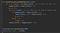Image 1: Insertion Sort Python Program to sort items in ascending order

Note that in the program above, I’ve tagged the lines in the function with numbers like ‘#1’, ‘#2’, ‘#3’ and so on .. (These are the ‘k’s — ‘the line numbers’-we’ll soon see it) so that the cost of a particular line ‘k’ can be looked up with the ‘cost image’ (Image 4) below.

Our task here is to measure the total number of steps executed by this program on that input(input_list). This is our measure of the ‘time cost’ incurred to run this code: We call it the Running Time. You hafta pretend the code above is a pseudo code, and machine independent — thank you! Now that it is done, assume the cost of executing ‘k’-th line once, as: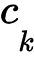Image 2: Assume the cost of executing the ‘k-th line once’ is this constant. We need to see how many times that line will be executed. Further, the total running time contributed by that line is simply, this cost multiplied by the number of times the line is executed.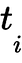Image 3: This represents the number of times the ‘for loop’ (at #4) would execute for that value of ‘i’ (‘i’ as shown in the program)

So, the total running time of the above algorithm will be sum of the possible running times of each of the lines shown. For lines 1 to 10, we can easily see that, the running times are (in the manner of cost for running once * total number if runs, line by line):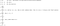Image 4. Costs incurred by line 1 to 10 (from top to bottom in that order). Here I used this link to create the mathematical symbols I needed.

The for loop executes one step more than its body does (i.e., The last check to leave the loop makes it one step extra than the body would).

The ‘else’ keyword at #8 might not incur a cost at all, although I showed a cost against the else keyword in #8. Anyway, the sum of all the items in the Image 4 is the cost of the entire function. So:Image 5. Generic Equation for Running time of the Given Insertion Sort

Now, Let’s see the Best Case running time: That would be the case where the input is already sorted in ascending order. So:

In the above situation, it will spend time in Line #1, #2 and #3. And remember that the input is sorted in ascending order already. So, soon after it enters the inner loop at #4, the check at #5 fails. The ‘else’ gets hit, and the ‘break’ at #9 gets hit. Thus the ‘for loop’ at #4 will be exited. i.e., upon each entry of the inner loop, this inner loop gets hurriedly exited upon the first check itself. So, time spent in #6 and #7 is zero. Finally, it spends some time at #10. Note that I avoided #8. Representing this into our simple mathematical notation:Image 6: Best Case Running time Equation — Needs simplification

Note that both the ‘for’ loop at #4 and its body execute equal number of times due to the ‘break’ in the body. Simplifying this:Image 7. Running time for the Best Case

This is Cn - C’ : So the best case is a humble linear function on input size ‘n’, where C and C’ are constants determined by the cost of individual statements.

But we know that the best case running time is never a guarantee of the algorithm’s overall performance: the worst case could be slower. So, here we are, finally about to find that real guarantee: The worst case running time. Spoiler alert: If you have visualised the program, it is actually easy to see that the worst case is of the order of n². That is, you can see for each iteration of the loop in #1, the second loop at #4 will execute to the ‘maximum’ number of times . That is like ‘n*n’, hence n².

Never mind, let’s take a detailed peek. The worst scenario happens when the input is already in the descending order: Now, #1, #2 and #3 execute as usual. The line at #4 gets executed. Then the check at #5 succeeds (will be true), and it will painfully loop from j = (i — 1) down to j = 0. (That is where it behaves differently from the best case) After that, it executes line #10. Note that the inner loop at #4 would execute in this pattern: first for index 0, then for indices 0 & 1, then for indices 0 & 1 & 2 .. and so on. That means, it will loop 1 time, then 2 times, then 3 times ….and so on upto ‘n-1’ times. That is to say, t1 = 1, t2 =2, t3 = 3, …. etc. So the total is ‘1 + 2 + 3 + …. +(n-1)’. Easy-peasy! Also note that in this case, the inner loop #4 loops one step more than its body does — for doing the last check. Representing all this in our simple mathematical lingo: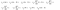Image 8. The ‘worst case’ Running Time Equation

(Note that line #4 loops one more than #5 and #6). Simplifying this:

This can be simplified to:

Where constants A, B, C are dependent on the costs of each line. This is a quadratic equation — our guarantee: The worst case running time for insertion sort.

## The Startup

Get smarter at building your thing. Join The Startup’s +788K followers.

## Sign up for Top 10 Stories

### By The Startup

Get smarter at building your thing. Subscribe to receive The Startup's top 10 most read stories — delivered straight into your inbox, once a week. Take a look.

By signing up, you will create a Medium account if you don’t already have one. Review our Privacy Policy for more information about our privacy practices.

Check your inbox
Medium sent you an email at to complete your subscription.

Written by

## Sunny Man

The man who forgot what to do

## The Startup

Get smarter at building your thing. Follow to join The Startup’s +8 million monthly readers & +788K followers.

Written by

## Sunny Man

The man who forgot what to do## The Startup

Get smarter at building your thing. Follow to join The Startup’s +8 million monthly readers & +788K followers.

## More From Medium

Medium is an open platform where 170 million readers come to find insightful and dynamic thinking. Here, expert and undiscovered voices alike dive into the heart of any topic and bring new ideas to the surface. Learn more

Follow the writers, publications, and topics that matter to you, and you’ll see them on your homepage and in your inbox. Explore

If you have a story to tell, knowledge to share, or a perspective to offer — welcome home. It’s easy and free to post your thinking on any topic. Write on Medium